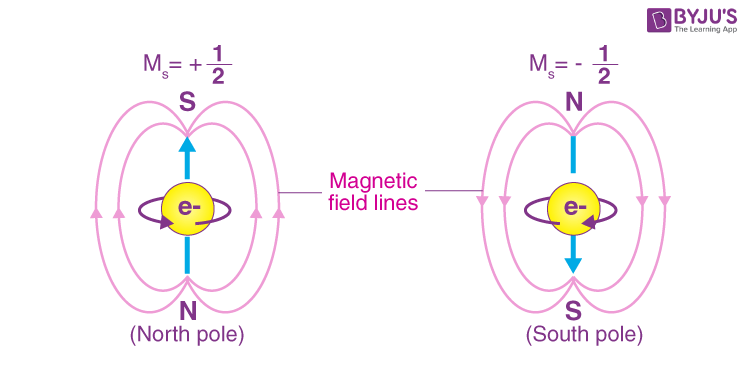# Electron Spin

S.A. Goudsmit and G.E. Uhlenbeck, in 1925, recommended that an electron has an inherent angular momentum that is a magnetic moment that is recognised as spin. In atomic physics, the inherent angular momentum of a particular particle is parametrized by spin quantum numbers. The spin quantum number is the fourth number. The rest three are a principal quantum number, azimuthal, and magnetic quantum number. The spin quantum number explains the unique quantum state of an electron. This is nominated as ‘s’.

The spins play a noteworthy role in quantum mechanics in computing the characteristics of elementary units like electrons. The spin direction of the particle regulates several things like the spin quantum number, angular momentum, the degree of freedom, etc. Let us learn more about the electron spin in this segment.

## What is Electron Spin?

The electron spin is one of the three inherent properties of the electrons; the others are the mass and charge of the electron. The electron spin is described as the electron spinning around its axis.

It is articulated as:

$$\begin{array}{l}\left \| S \right \| = \sqrt{s(s+1)h}\end{array}$$

Where,

• s is equivalent to a quantized spin vector.
• The spin vector is articulated as ||s||.
• The spin quantum number (s) is associated with the spin angular momentum and h is Planck’s constant.

### The spin quantum number can be articulated as:

$$\begin{array}{l}S = \frac{n}{2}\end{array}$$

Any non-negative integer can be n.
The permitted values of the spins are 0, 1/2, 1, 3/2, 2, etc.
The intrinsic angular momentum of the Electron is signified by quantum number 1/2

The total angular momentum s is articulated by:

$$\begin{array}{l}s = \sqrt{n(n+1)h}\end{array}$$
$$\begin{array}{l}s = \sqrt{\frac{1}{2}(\frac{1}{2}+1)h}\end{array}$$

$$\begin{array}{l}S = \sqrt{\frac{3}{4}\bar{h}}\end{array}$$

Where,
the reduced Planck’s constant is ℏ
ℏ = h/2π.

## Electron Spin Theory

As in classical theory, the electron spin theory describes the electron as a quantum particle instead of a simple sphere.

The theory says that “the electron spin direction and its influence on certain properties like the atom’s magnetic properties”.### The electron can spin in two directions:

1. Spin up
2. Spin down

The spin up and spin down directions correspond to the spinning in the “+z” or “–z” direction. These spins (spin up and spin down) are the particles that have spin “s” equal to 1/2, i.e. for electrons.

In quantum theory, the electron is thought of as the minute magnetic bar, and its spin points to the north pole of the minute bar. If two proximate electrons have a similar spin direction, the magnetic field formed by them strengthens each other, and therefore a strong magnetic field is gained. If the proximate electrons have an opposite spin direction, the magnetic field formed by them cancels each other, and no magnetic field is existent.

## Watch the video and understand the rules of electronic configuration.## Frequently Asked Questions – FAQs

Q1

### What are the three inherent properties of the electrons?

Three inherent properties of the electrons are:
• Mass
• Charge of the electron
• Electron spin
Q2

### What are the two directions in which an electron spins?

Electron spins in two directions:
• Spin up
• Spin down
Q3

### What is the formula to calculate the spin quantum number?

The spin quantum number is given by the formula:
$$\begin{array}{l}S = \frac{n}{2} .\end{array}$$
Q4

### What is the formula to calculate the electron spin?

Electron spin is given by the formula:

$$\begin{array}{l}\left \| S \right \| = \sqrt{s(s+1)h}.\end{array}$$
Q5

### State true or false: The spin quantum number explains the unique quantum state of an electron.

True.

Stay tuned to BYJU’S and Fall in Love with Learning!

Test Your Knowledge On Electron Spin!